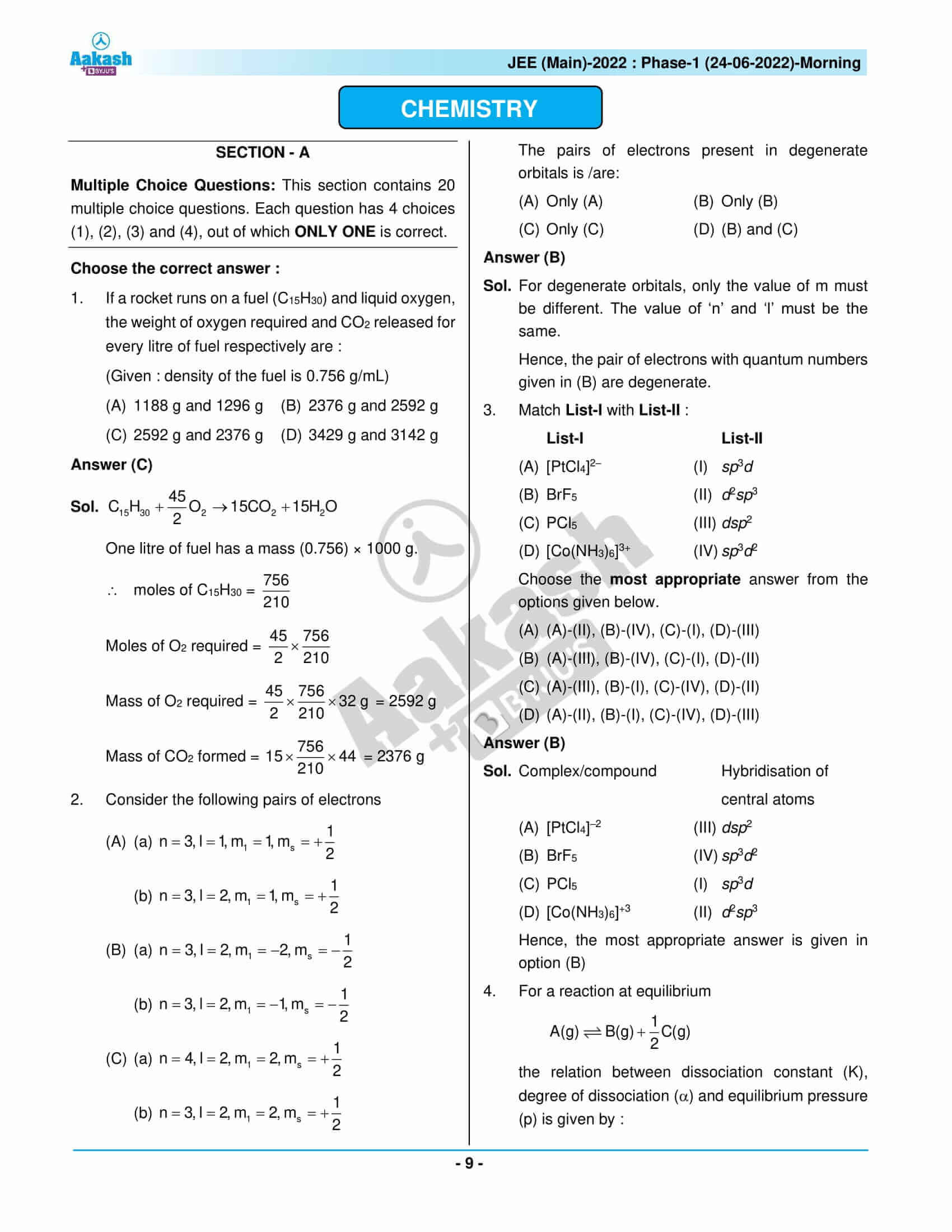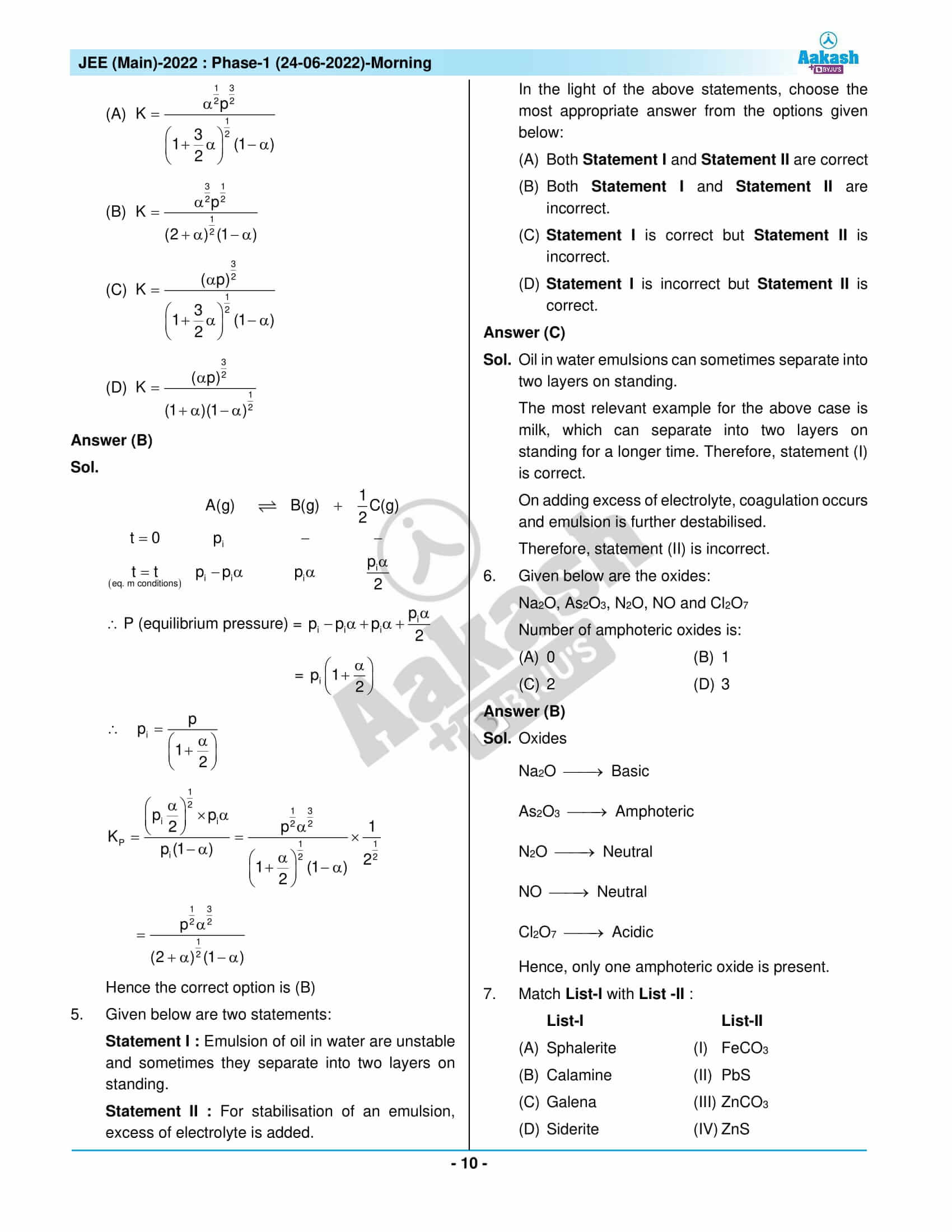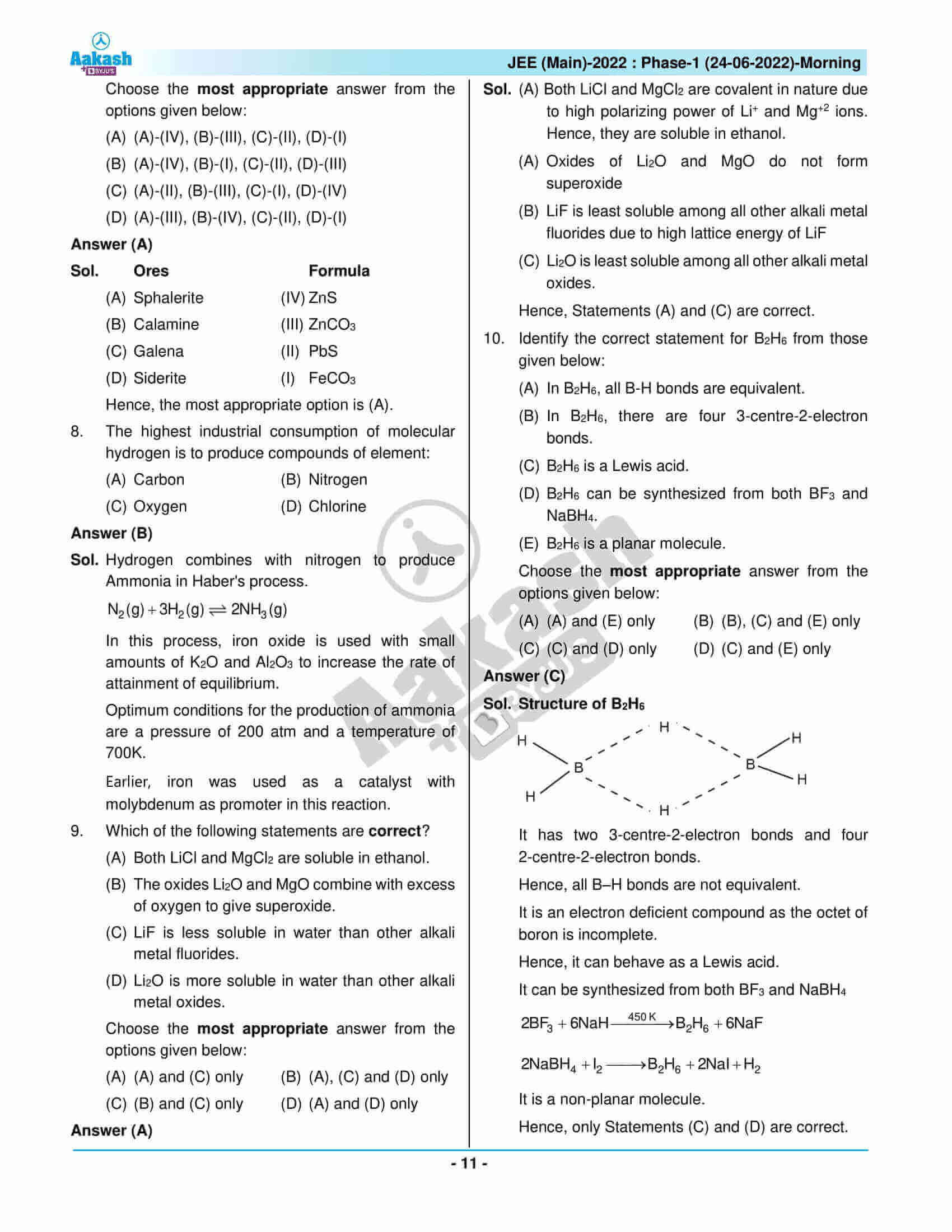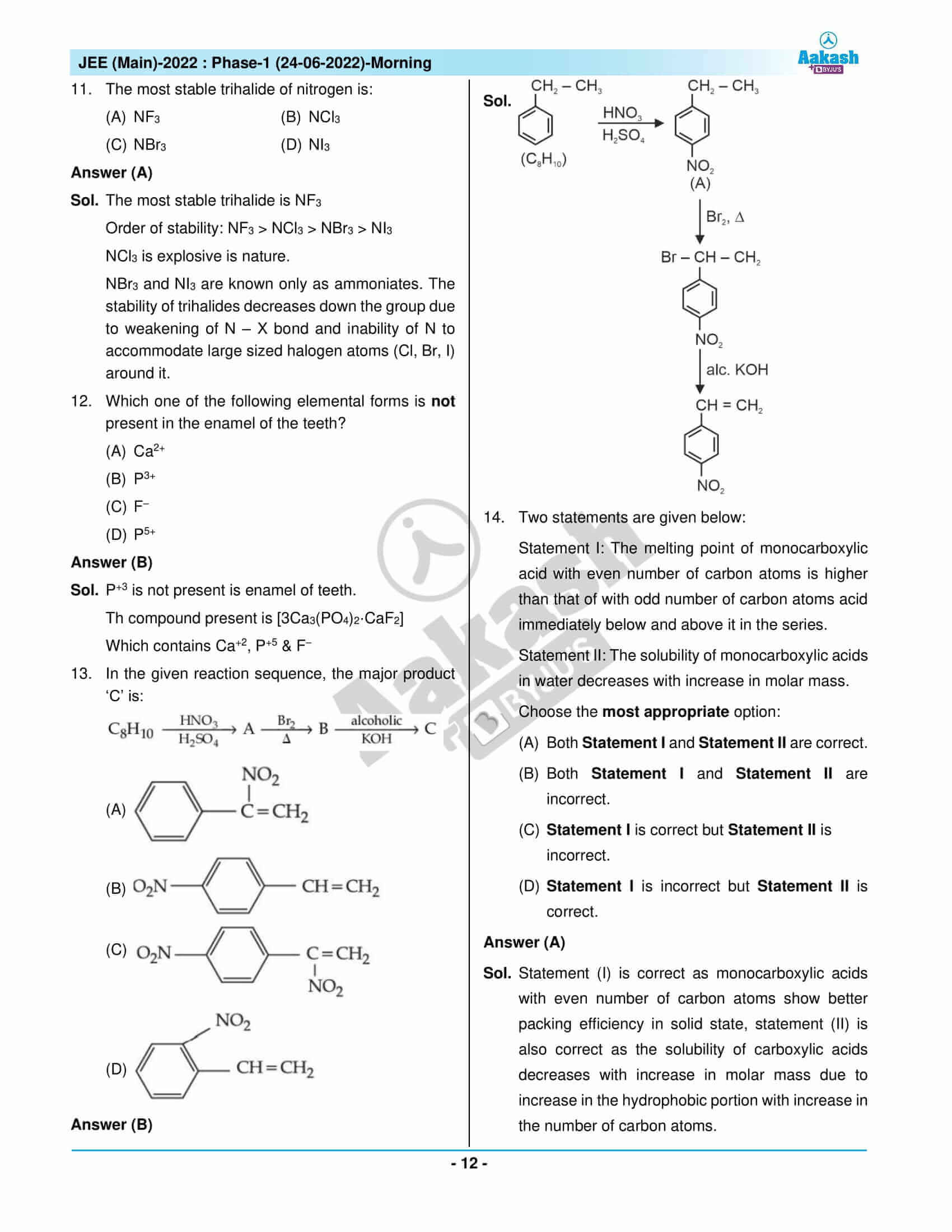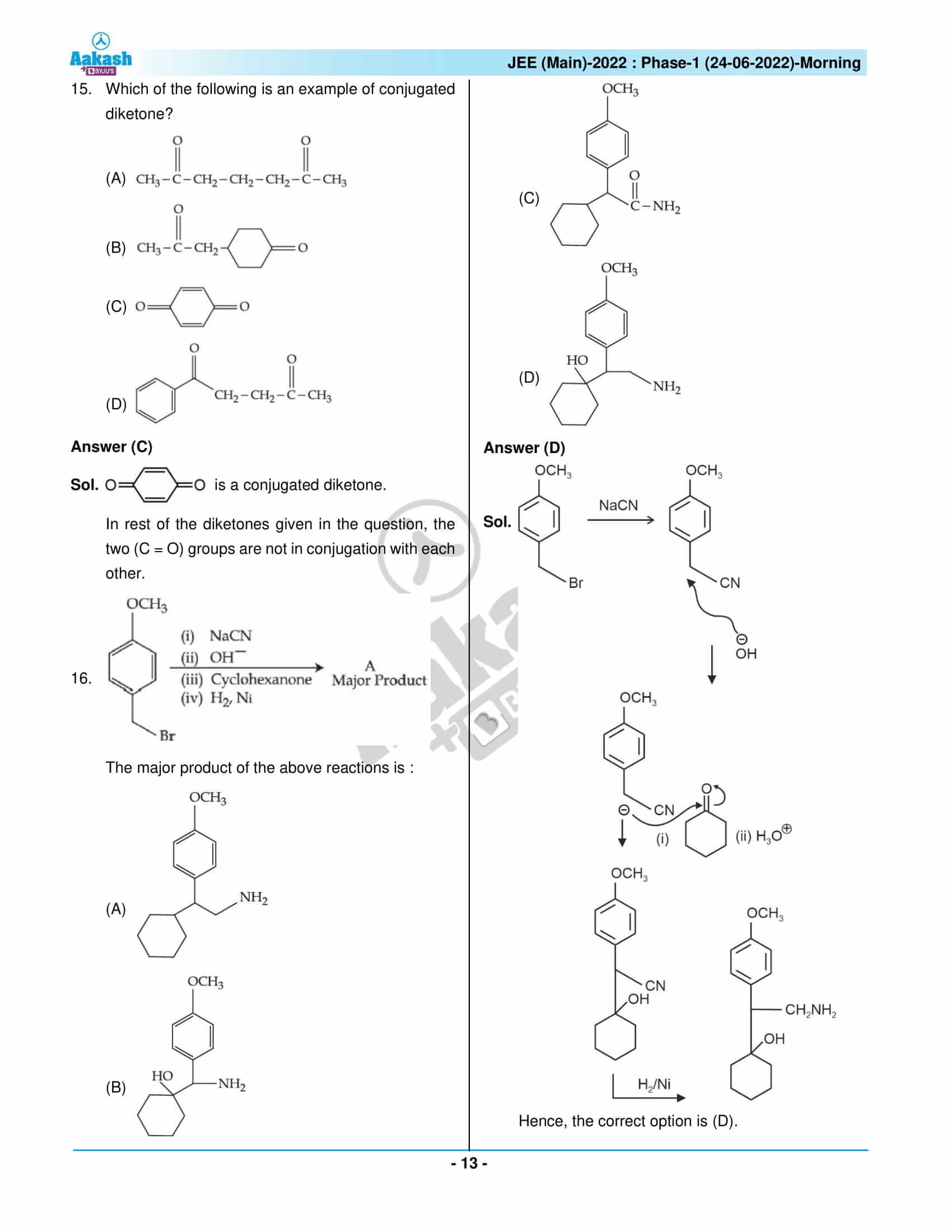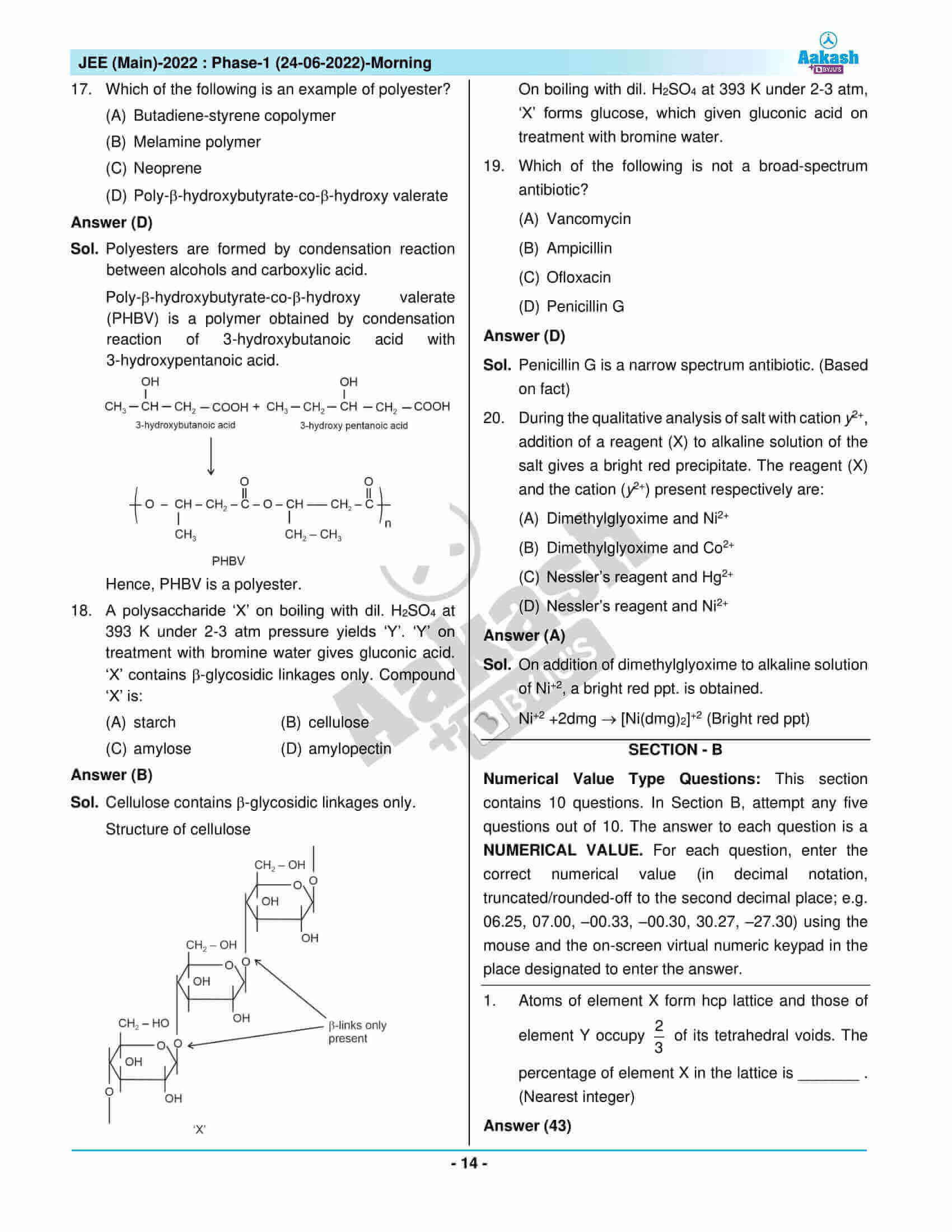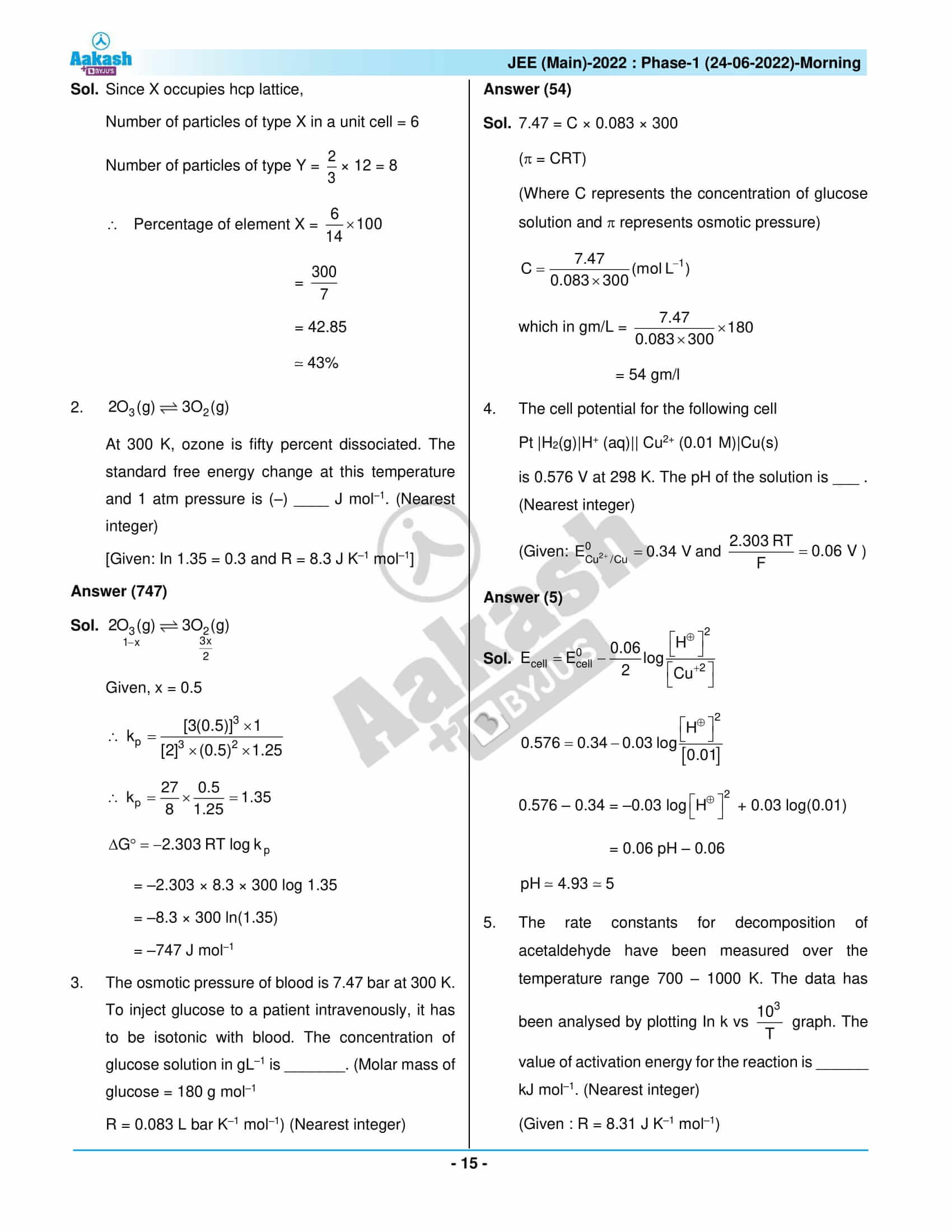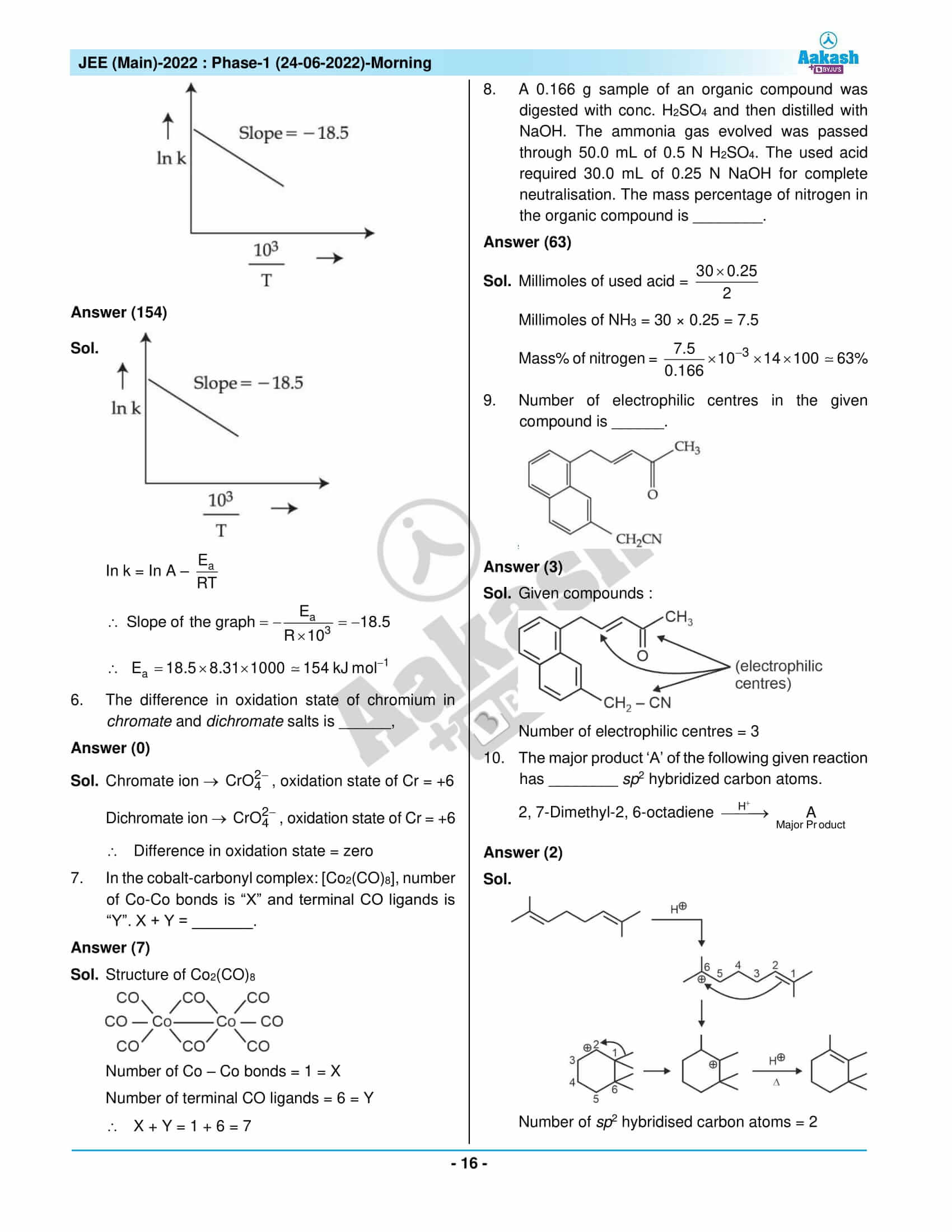Win up to 100% scholarship on Aakash BYJU'S JEE/NEET courses with ABNAT Win up to 100% scholarship on Aakash BYJU'S JEE/NEET courses with ABNAT

# JEE Main 2022 June 24 – Shift 1 Chemistry Question Paper with Solutions

JEE Main 2022 June 24 – Shift 1 Chemistry Question Paper with Solutions

SECTION – A

Multiple Choice Questions: This section contains 20 multiple choice questions. Each question has 4 choices (1), (2), (3) and (4), out of which ONLY ONE is correct.

1. If a rocket runs on a fuel (C15H30) and liquid oxygen, the weight of oxygen required and CO2 released for every litre of fuel respectively are :

(Given : density of the fuel is 0.756 g/mL)

(A) 1188 g and 1296 g

(B) 2376 g and 2592 g

(C) 2592 g and 2376 g

(D) 3429 g and 3142 g

Sol.

$$\begin{array}{l}C_{15}H_{30}+\frac{45}{2}O_2\rightarrow15CO_2+15H_2O\end{array}$$

One litre of fuel has a mass (0.756) × 1000 g.

∴ moles of C15H30 =

$$\begin{array}{l}\frac{756}{210}\end{array}$$

Moles of O2 required =

$$\begin{array}{l}\frac{45}{2}\times\frac{756}{210}\end{array}$$

Mass of O2 required =

$$\begin{array}{l}\frac{45}{2}\times\frac{756}{210}\times32\text{ g}=2592 \text{ g}\end{array}$$

Mass of CO2 formed =

$$\begin{array}{l}15\times\frac{756}{210}\times 44=2376 \text{ g}\end{array}$$

2. Consider the following pairs of electronsstrong>

(A)

$$\begin{array}{l}\left ( a \right )n=3, l = 1, m_1 = 1, m_s=+\frac{1}{2}\end{array}$$
$$\begin{array}{l}\left ( b \right )n=3, l = 2, m_1 = 1, m_s=+\frac{1}{2}\end{array}$$

(B)

$$\begin{array}{l}\left ( a \right )n=3, l = 2, m_1 = -2, m_s=-\frac{1}{2}\end{array}$$
$$\begin{array}{l}\left ( b \right )n=3, l = 2, m_1 = -1, m_s=-\frac{1}{2}\end{array}$$

(C)

$$\begin{array}{l}\left ( a \right )n=4, l = 2, m_1 = 2, m_s=+\frac{1}{2}\end{array}$$
$$\begin{array}{l}\left ( b \right )n=3, l = 2, m_1 = 2, m_s=+\frac{1}{2}\end{array}$$

The pairs of electrons present in degenerate orbitals is /are:

(A) Only (A)

(B) Only (B)

(C) Only (C)

(D) (B) and (C)

Sol. For degenerate orbitals, only the value of m must be different. The value of ‘n’ and ‘l’ must be the same.

Hence, the pair of electrons with quantum numbers given in (B) are degenerate.

3. Match List-I with List-II :

List-I

List-II

(A)

[PtCl4]2–

(I)

sp3d

(B)

BrF5

(II)

d2sp3

(C)

PCl5

(III)

dsp2

(D)

[Co(NH3)6]3+

(IV)

sp3d2

Choose the most appropriate answer from the options given below.

(A) (A)-(II), (B)-(IV), (C)-(I), (D)-(III)

(B) (A)-(III), (B)-(IV), (C)-(I), (D)-(II)

(C) (A)-(III), (B)-(I), (C)-(IV), (D)-(II)

(D) (A)-(II), (B)-(I), (C)-(IV), (D)-(III)

Sol.

Complex/compound

Hybridisation of central atoms

(A)

[PtCl4]–2

(III)

dsp2

(B)

BrF5

(IV)

sp3d2

(C)

PCl5

(I)

sp3d

(D)

[Co(NH3)6]+3

(II)

d2sp3

Hence, the most appropriate answer is given in option (B)

4. For a reaction at equilibrium

$$\begin{array}{l}A\left ( g \right )\rightleftharpoons B\left ( g \right )+\frac{1}{2}\left ( g \right )\end{array}$$

the relation between dissociation constant (K), degree of dissociation (α) and equilibrium pressure (p) is given by :

(A)

$$\begin{array}{l}K=\frac{\alpha^{\frac{1}{2}}p^{\frac{3}{2}}}{\left ( 1+\frac{3}{2}\alpha \right )^{\frac{1}{2}}\left ( 1-\alpha \right )}\end{array}$$

(B)

$$\begin{array}{l}K=\frac{\alpha^{\frac{3}{2}}p^{\frac{1}{2}}}{\left ( 2+\alpha \right )^{\frac{1}{2}}\left ( 1-\alpha \right )}\end{array}$$

(C)

$$\begin{array}{l}K=\frac{\left ( \alpha p \right )^{\frac{3}{2}}}{\left ( 1+\frac{3}{2}\alpha \right )^{\frac{1}{2}}\left ( 1-\alpha \right )}\end{array}$$

(D)

$$\begin{array}{l}K=\frac{\left ( \alpha p \right )^{\frac{3}{2}}}{\left ( 1+\alpha \right )\left ( 1-\alpha \right )^{\frac{1}{2}}}\end{array}$$

Sol.

$$\begin{array}{l}\begin{matrix} & A(g) & \rightleftharpoons & B(g) & + & \frac{1}{2}C(g) \\ t=0 &p_i & & – & & – \\\underset{\text{eq. m conditons}}{t=t} & p_i – P_i\alpha & & p_i\alpha & & \frac{p_i\alpha}{2} \\\end{matrix}\end{array}$$

∴ P (equilibrium pressure) =

$$\begin{array}{l}p_i-p_i\alpha+p_i\alpha+\frac{p_i\alpha}{2}\end{array}$$
$$\begin{array}{l}=p_i\left ( 1+ \frac{\alpha}{2} \right )\end{array}$$
$$\begin{array}{l}\therefore p_i=\frac{p}{\left ( 1+\frac{\alpha}{2} \right )}\end{array}$$
$$\begin{array}{l}K_p=\frac{\left ( p_i\frac{\alpha}{2} \right )^{\frac{1}{2}}\times p_i\alpha}{p_i\left ( 1-\alpha \right )}=\frac{p^{\frac{1}{2}}\alpha^{\frac{3}{2}}}{\left ( 1+\frac{\alpha}{2} \right )^{\frac{1}{2}}\left ( 1-\alpha \right )}\times\frac{1}{2^{\frac{1}{2}}}\end{array}$$
$$\begin{array}{l}=\frac{p^{\frac{1}{2}}\alpha^{\frac{3}{2}}}{\left ( 2+\alpha \right )^{\frac{1}{2}}\left ( 1-\alpha \right )}\end{array}$$

Hence the correct option is (B).

5.Given below are two statements:

Statement I : Emulsion of oil in water are unstable and sometimes they separate into two layers on standing.

Statement II : For stabilisation of an emulsion, excess of electrolyte is added.

In the light of the above statements, choose the most appropriate answer from the options given below:

(A) Both Statement I and Statement II are correct

(B) Both Statement I and Statement II are incorrect.

(C) Statement I is correct but Statement II is incorrect.

(D) Statement I is incorrect but Statement II is correct.

Sol. Oil in water emulsions can sometimes separate into two layers on standing.

The most relevant example for the above case is milk, which can separate into two layers on standing for a longer time. Therefore, statement (I) is correct.

On adding excess of electrolyte, coagulation occurs and emulsion is further destabilised.

Therefore, statement (II) is incorrect.

6. Given below are the oxides:

Na2O, As2O3, N2O, NO and Cl2O7

Number of amphoteric oxides is:

(A) 0

(B) 1

(C) 2

(D) 3

Sol. Oxides

$$\begin{array}{l}Na_2O\rightarrow \text{Basic}\end{array}$$
$$\begin{array}{l}As_2O_3\rightarrow \text{Amphoteric}\end{array}$$
$$\begin{array}{l}N_2O\rightarrow \text{Neutral}\end{array}$$
$$\begin{array}{l}NO\rightarrow \text{Neutral}\end{array}$$
$$\begin{array}{l}Cl_2O_7\rightarrow \text{Acidic}\end{array}$$

Hence, only one amphoteric oxide is present.

7. Match List-I with List -II :

List-I

List-II

(A)

Sphalerite

(I)

FeCO3

(B)

Calamine

(II)

PbS

(C)

Galena

(III)

ZnCO3

(D)

Siderite

(IV)

ZnS

Choose the most appropriate answer from the options given below:

(A) (A)-(IV), (B)-(III), (C)-(II), (D)-(I)

(B) (A)-(IV), (B)-(I), (C)-(II), (D)-(III)

(C) (A)-(II), (B)-(III), (C)-(I), (D)-(IV)

(D) (A)-(III), (B)-(IV), (C)-(II), (D)-(I)

Sol.

Ores

Formula

(A)

Sphalerite

(IV)

ZnS

(B)

Calamine

(III)

ZnCO3

(C)

Galena

(II)

PbS

(D)

Siderite

(I)

FeCO3

Hence, the most appropriate option is (A).

8. The highest industrial consumption of molecular hydrogen is to produce compounds of element:

(A) Carbon

(B) Nitrogen

(C) Oxygen

(D) Chlorine

Sol. Hydrogen combines with nitrogen to produce Ammonia in Haber’s process.

$$\begin{array}{l}N_2\left ( g \right )+3H_2\left ( g \right )\rightleftharpoons 2NH_3\left ( g \right )\end{array}$$

In this process, iron oxide is used with small amounts of K2O and Al2O3 to increase the rate of attainment of equilibrium.

Optimum conditions for the production of ammonia are a pressure of 200 atm and a temperature of 700K.

Earlier, iron was used as a catalyst with molybdenum as promoter in this reaction.

9. Which of the following statements are correct?

(A) Both LiCl and MgCl2 are soluble in ethanol.

(B) The oxides Li2O and MgO combine with excess of oxygen to give superoxide.

(C) LiF is less soluble in water than other alkali metal fluorides.

(D) Li2O is more soluble in water than other alkali metal oxides.

Choose the most appropriate answer from the options given below:

(A) (A) and (C) only

(B) (A), (C) and (D) only

(C) (B) and (C) only

(D) (A) and (D) only

Sol. (A) Both LiCl and MgCl2 are covalent in nature due to high polarizing power of Li+ and Mg+2 ions. Hence, they are soluble in ethanol.

1. Oxides of Li2O and MgO do not form superoxide
2. LiF is least soluble among all other alkali metal fluorides due to high lattice energy of LiF
3. Li2O is least soluble among all other alkali metal oxides.

Hence, Statements (A) and (C) are correct.

10. Identify the correct statement for B2H6 from those given below:

(A) In B2H6, all B-H bonds are equivalent.

(B) In B2H6, there are four 3-centre-2-electron bonds.

(C) B2H6 is a Lewis acid.

(D) B2H6 can be synthesized from both BF3 and NaBH4.

(E) B2H6 is a planar molecule.

Choose the most appropriate answer from the options given below:

(A) (A) and (E) only

(B) (B), (C) and (E) only

(C) (C) and (D) only

(D) (C) and (E) only

Sol. Structure of B2H6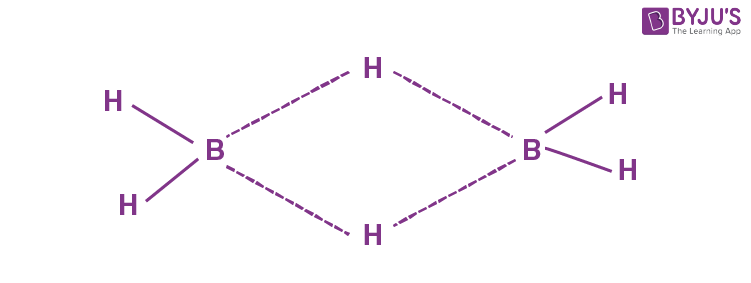It has two 3-centre-2-electron bonds and four
2-centre-2-electron bonds.

Hence, all B–H bonds are not equivalent.

It is an electron deficient compound as the octet of boron is incomplete.

Hence, it can behave as a Lewis acid.

It can be synthesized from both BF3 and NaBH4

$$\begin{array}{l}2BF_3+6NaH \xrightarrow{~~450 K~~} B_2H_6+6NaF \end{array}$$
$$\begin{array}{l}2NaBH_4+I_2\longrightarrow B_2H_6+2NaI + H_2\end{array}$$

It is a non-planar molecule.

Hence, only Statements (C) and (D) are correct.

11. The most stable trihalide of nitrogen is:

(A) NF3

(B) NCl3

(C) NBr3

(D) NI3

Sol. The most stable trihalide is NF3

Order of stability: NF3 > NCl3 > NBr3 > NI3

NCl3 is explosive is nature.

NBr3 and NI3 are known only as ammoniates. The stability of trihalides decreases down the group due to weakening of N – X bond and inability of N to accommodate large sized halogen atoms (Cl, Br, I) around it.

12. Which one of the following elemental forms is not present in the enamel of the teeth?

(A) Ca2+

(B) P3+

(C) F

(D) P5+

Sol. P+3 is not present is enamel of teeth.

Th compound present is [3Ca3(PO4)2·CaF2]

Which contains Ca+2, P+5 & F

13. In the given reaction sequence, the major product ‘C’ is:

$$\begin{array}{l}C_8H_{10} \overset{HNO_3}{\underset{H_2SO_4}\longrightarrow} A \overset{Br_2}{\underset{\Delta}\longrightarrow} B \overset{\text{alcoholic}}{\underset{KOH}\longrightarrow} C\end{array}$$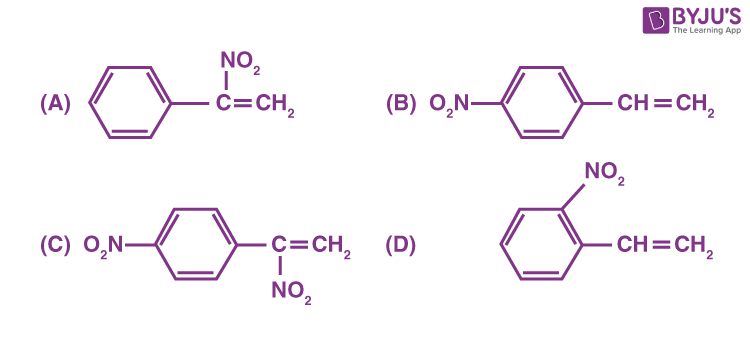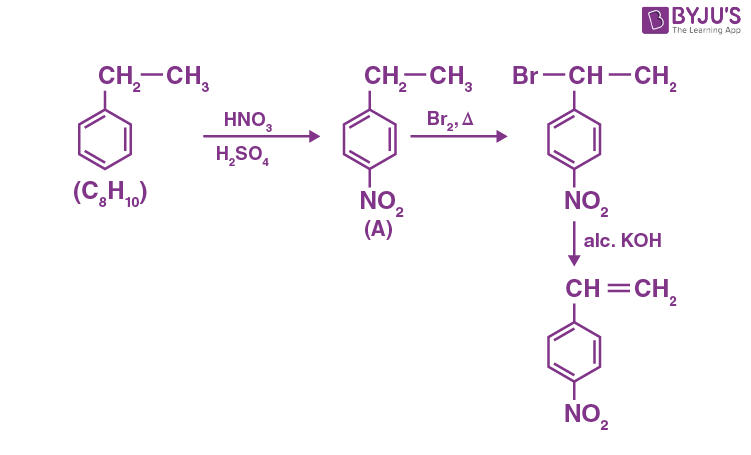Sol.

14. Two statements are given below:

Statement I: The melting point of monocarboxylic acid with even number of carbon atoms is higher than that of with odd number of carbon atoms acid immediately below and above it in the series.

Statement II: The solubility of monocarboxylic acids in water decreases with increase in molar mass.

Choose the most appropriate option:

(A) Both Statement I and Statement II are correct.

(B) Both Statement I and Statement II are incorrect.

(C) Statement I is correct but Statement II is incorrect.

(D) Statement I is incorrect but Statement II is correct.

Sol. Statement (I) is correct as monocarboxylic acids with even number of carbon atoms show better packing efficiency in solid state, statement (II) is also correct as the solubility of carboxylic acids decreases with increase in molar mass due to increase in the hydrophobic portion with increase in the number of carbon atoms.

15. Which of the following is an example of conjugated diketone?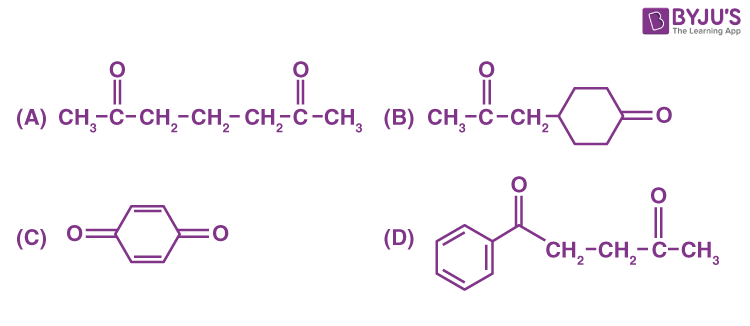Sol.is a conjugated diketone.

In rest of the diketones given in the question, the two (C = O) groups are not in conjugation with each other.

16.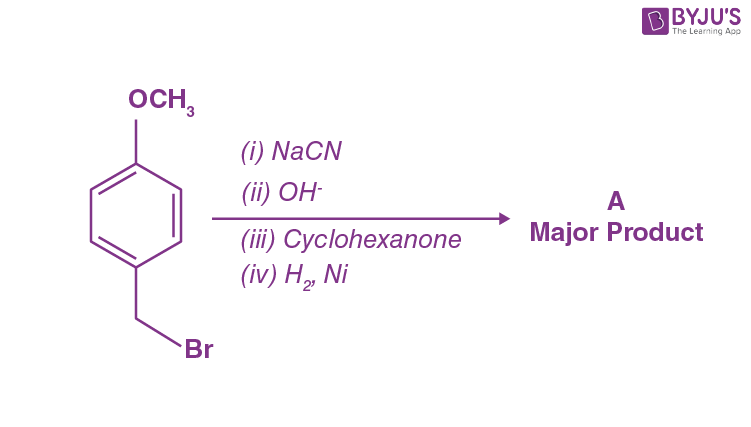The major product of the above reactions is :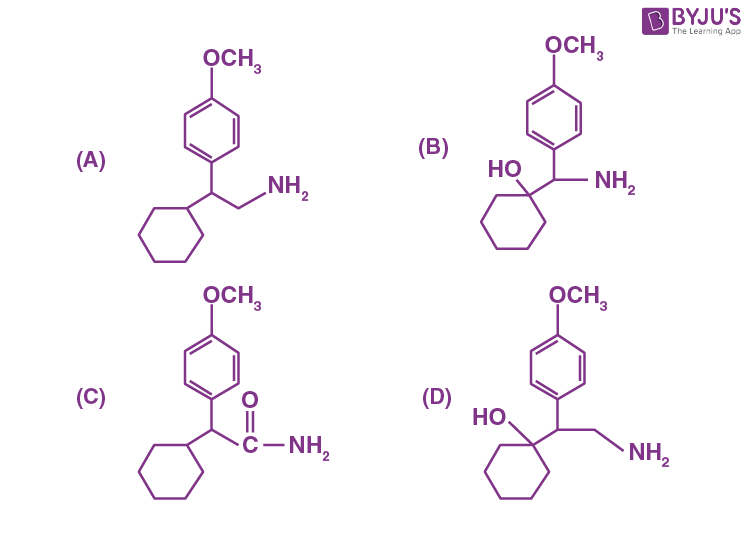Sol.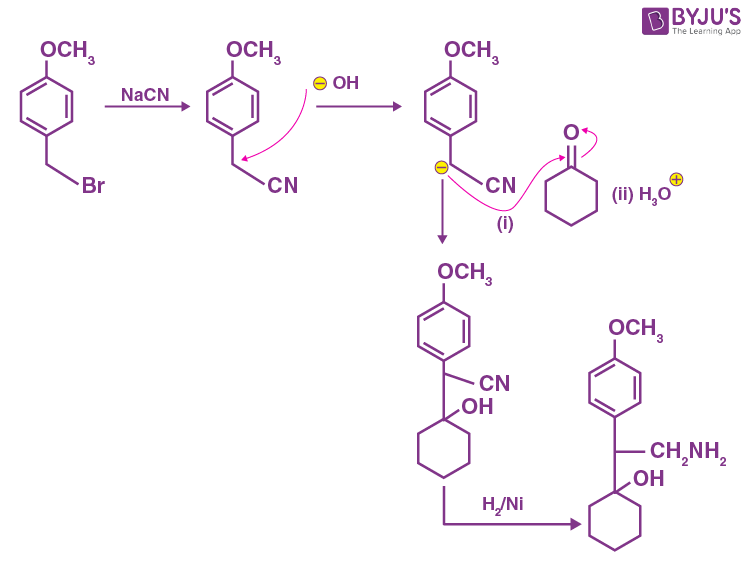Hence, the correct option is (D).

17. Which of the following is an example of polyester?

(B) Melamine polymer

(C) Neoprene

(D) Poly-β-hydroxybutyrate-co-β-hydroxy valerate

Sol. Polyesters are formed by condensation reaction between alcohols and carboxylic acid.

Poly-β-hydroxybutyrate-co-β-hydroxy valerate (PHBV) is a polymer obtained by condensation reaction of 3-hydroxybutanoic acid with 3-hydroxypentanoic acid.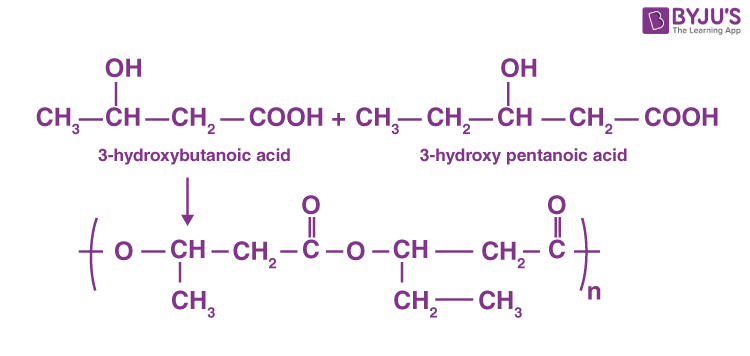Hence, PHBV is a polyester.

18. A polysaccharide ‘X’ on boiling with dil. H2SO4 at 393 K under 2-3 atm pressure yields ‘Y’. ‘Y’ on treatment with bromine water gives gluconic acid. ‘X’ contains β-glycosidic linkages only. Compound ‘X’ is:

(A) starch

(B) cellulose

(C) amylose

(D) amylopectin

Sol. Cellulose contains β-glycosidic linkages only.

Structure of cellulose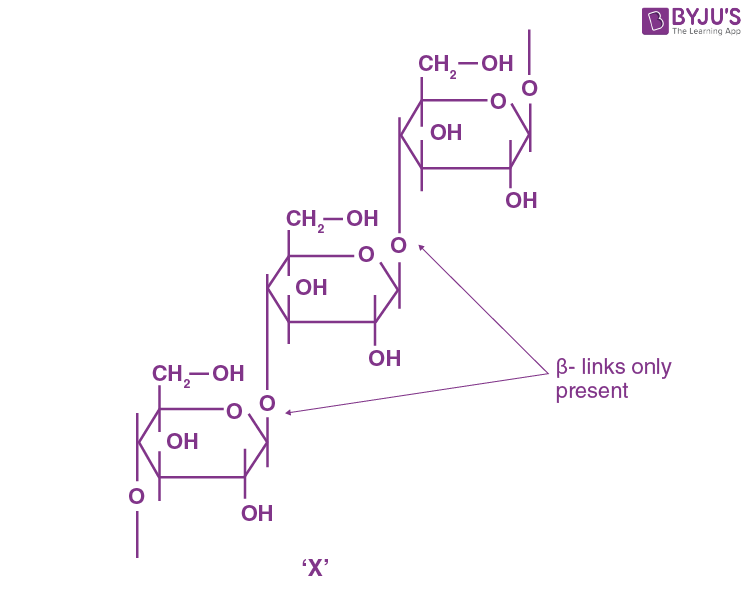On boiling with dil. H2SO4 at 393 K under 2-3 atm, ‘X’ forms glucose, which given gluconic acid on treatment with bromine water.

19. Which of the following is not a broad-spectrum antibiotic?

(A) Vancomycin

(B) Ampicillin

(C) Ofloxacin

(D) Penicillin G

Sol. Penicillin G is a narrow spectrum antibiotic. (Based on fact)

20. During the qualitative analysis of salt with cation y2+, addition of a reagent (X) to alkaline solution of the salt gives a bright red precipitate. The reagent (X) and the cation (y2+) present respectively are:

(A) Dimethylglyoxime and Ni2+

(B) Dimethylglyoxime and Co2+

(C) Nessler’s reagent and Hg2+

(D) Nessler’s reagent and Ni2+

Sol. On addition of dimethylglyoxime to alkaline solution of Ni+2, a bright red ppt. is obtained.

Ni+2 +2dmg → [Ni(dmg)2]+2 (Bright red ppt)

SECTION – B

Numerical Value Type Questions: This section contains 10 questions. In Section B, attempt any five questions out of 10. The answer to each question is a NUMERICAL VALUE. For each question, enter the correct numerical value (in decimal notation, truncated/rounded-off to the second decimal place; e.g. 06.25, 07.00, –00.33, –00.30, 30.27, –27.30) using the mouse and the on-screen virtual numeric keypad in the place designated to enter the answer.

1. Atoms of element X form hcp lattice and those of element Y occupy

$$\begin{array}{l}\frac{2}{3}\end{array}$$
of its tetrahedral voids. The percentage of element X in the lattice is _______ . (Nearest integer)

Sol. Since X occupies hcp lattice,

Number of particles of type X in a unit cell = 6

Number of particles of type

$$\begin{array}{l}Y=\frac{2}{3}\times 12=8\end{array}$$

∴Percentage of element

$$\begin{array}{l}Y=\frac{6}{14}\times 100\end{array}$$
$$\begin{array}{l}=\frac{300}{7}\end{array}$$

= 42.85

= 43% (approx)

2.

$$\begin{array}{l}2O_3(g)\rightleftharpoons 3O_2(g)\end{array}$$

At 300 K, ozone is fifty percent dissociated. The standard free energy change at this temperature and 1 atm pressure is (–) ____ J mol–1. (Nearest integer)

[Given: In 1.35 = 0.3 and R = 8.3 J K–1 mol–1]

Sol.

$$\begin{array}{l}\underset{1-x}{2O_3}(g)\rightleftharpoons \underset{\frac{3x}{2}}{3O_2} (g)\end{array}$$

Given, x = 0.5

$$\begin{array}{l}\therefore k_p=\frac{\left [ 3\left ( 0.5 \right ) \right ]^3\times 1}{\left [ 2 \right ]^3\times\left ( 0.5 \right )^2\times1.25}\end{array}$$
$$\begin{array}{l}\therefore k_p=\frac{27}{8}\times\frac{0.5}{1.25}=1.35\end{array}$$
$$\begin{array}{l}\Delta G^{\circ} = -2.303 \text{RT log k}_\text{p}\end{array}$$

= –2.303 × 8.3 × 300 log 1.35

= –8.3 × 300 ln(1.35)

= –747 J mol–1

3. The osmotic pressure of blood is 7.47 bar at 300 K. To inject glucose to a patient intravenously, it has to be isotonic with blood. The concentration of glucose solution in gL–1 is _______. (Molar mass of glucose = 180 g mol–1

R = 0.083 L bar K–1 mol–1) (Nearest integer)

Sol. 7.47 = C × 0.083 × 300

(π = CRT)

(Where C represents the concentration of glucose solution and π represents osmotic pressure)

$$\begin{array}{l}C=\frac{7.47}{0.083\times 300}\left ( \text{molL}^{-1} \right )\end{array}$$

which in gm/L

$$\begin{array}{l}\frac{7.47}{0.083\times 300}\times 80\end{array}$$

= 54 gm/l

4. The cell potential for the following cell

Pt |H2(g)|H+ (aq)|| Cu2+ (0.01 M)|Cu(s)

is 0.576 V at 298 K. The pH of the solution is ___ . (Nearest integer)

$$\begin{array}{l}\left ( \text{Given: }E_{Cu^{2+}/Cu}^0 =0.34 \text{ V and } \frac{2.303 RT}{F}=0.06 \text{ V}\right )\end{array}$$

Sol.

$$\begin{array}{l}E_{cell}=E_{cell}^0-\frac{0.06}{2}\log\frac{\left [ H^{\oplus} \right ]^2}{\left [ Cu^{+2} \right ]}\end{array}$$
$$\begin{array}{l}0.576=0.034-0.03 \log\frac{\left [ H^{\oplus} \right ]^2}{\left [ 0.01 \right ]} \end{array}$$
$$\begin{array}{l}0.576-0.34=-0.03\log\left [ H^{\oplus} \right ]^2+0.03\log\left ( 0.01 \right )\end{array}$$

= 0.06 pH – 0.06

pH ≃ 4.93 ≃ 5

5. The rate constants for decomposition of acetaldehyde have been measured over the temperature range 700 – 1000 K. The data has been analysed by plotting In k vs

$$\begin{array}{l}\frac{10^3}{T}\end{array}$$
graph. The value of activation energy for the reaction is ______ kJ mol–1. (Nearest integer)

(Given : R = 8.31 J K–1 mol–1)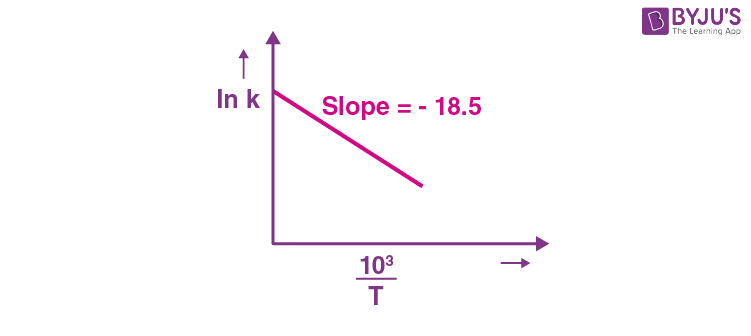Sol.$$\begin{array}{l}\ln k = \ln A-\frac{E_a}{RT}\end{array}$$
$$\begin{array}{l}\therefore \text{Slope of the graph}=-\frac{E_a}{R\times 10^3}=-18.5\end{array}$$
$$\begin{array}{l}\therefore E_a = 18.5 \times 8.31 \times 1000 \simeq 154 \text{ kJ mol}^{-1}\end{array}$$

6. The difference in oxidation state of chromium in chromate and dichromate salts is ______,

Sol. Chromate ion

$$\begin{array}{l}\rightarrow CrO_4^{2-}\end{array}$$
, oxidation state of Cr = +6

Dichromate ion

$$\begin{array}{l}\rightarrow CrO_4^{2-}\end{array}$$
, oxidation state of Cr = +6

∴ Difference in oxidation state = zero

7. In the cobalt-carbonyl complex: [Co2(CO)8], number of Co-Co bonds is “X” and terminal CO ligands is “Y”. X + Y = _______.

Sol. Structure of Co2(CO)8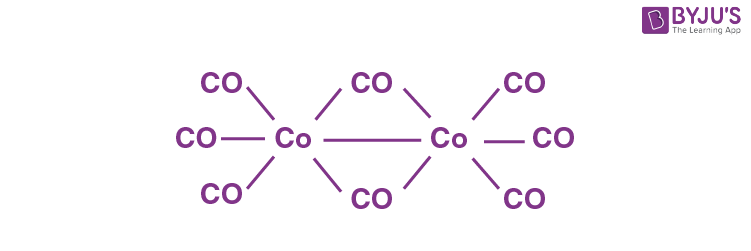Number of Co – Co bonds = 1 = X

Number of terminal CO ligands = 6 = Y

∴ X + Y = 1 + 6 = 7

8. A 0.166 g sample of an organic compound was digested with conc. H2SO4 and then distilled with NaOH. The ammonia gas evolved was passed through 50.0 mL of 0.5 N H2SO4. The used acid required 30.0 mL of 0.25 N NaOH for complete neutralisation. The mass percentage of nitrogen in the organic compound is ________.

Sol. Millimoles of used acid

$$\begin{array}{l}=\frac{30\times0.25}{2}\end{array}$$

Millimoles of NH3 = 30 × 0.25 = 7.5

Mass% of nitrogen

$$\begin{array}{l}=\frac{7.5}{0.166}\times 10^{-3}\times 14 \times 100 \simeq 63%\end{array}$$

9. Number of electrophilic centres in the given compound is ______.Sol. Given compounds :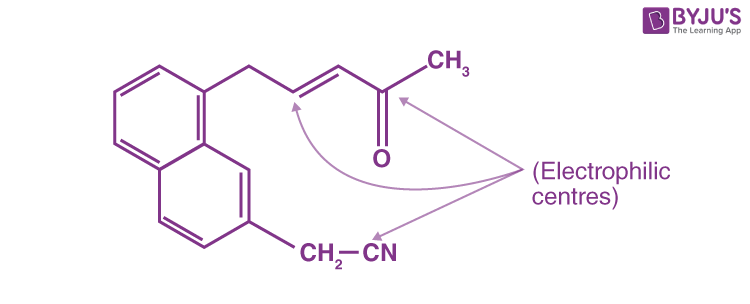Number of electrophilic centres = 3

10. The major product ‘A’ of the following given reaction has ________ sp2 hybridized carbon atoms.

$$\begin{array}{l}\overset{H^+}{{\longrightarrow}}\underset{\text{ Major Product}}{ A} \end{array}$$

Sol.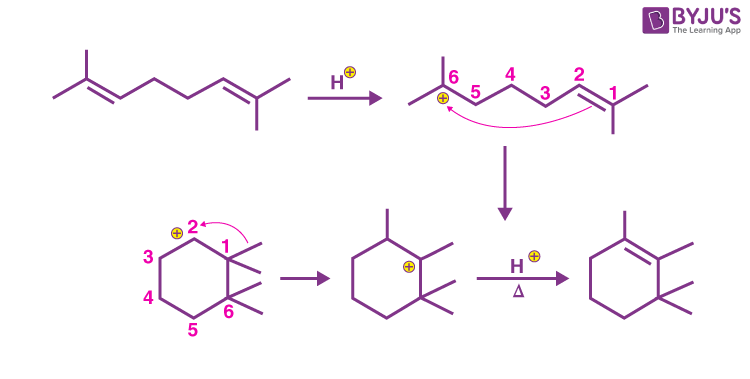Number of sp2 hybridised carbon atoms = 2